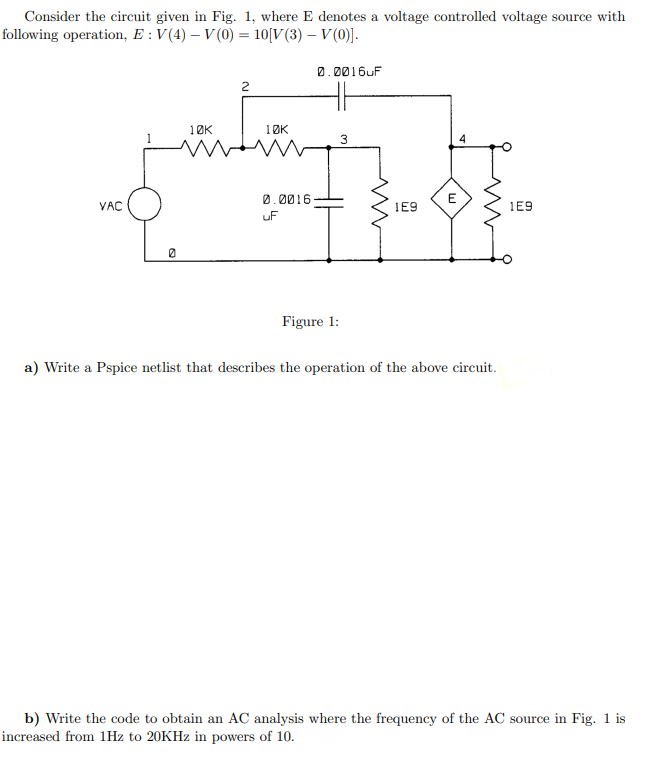# (Solved): i think question is crystal clear i need answer by using pspice   Consider the circuit give ...

i think question is crystal clear i need answer by using pspiceConsider the circuit given in Fig. 1, where E denotes a voltage controlled voltage source with following operation, $$E: V(4)-V(0)=10[V(3)-V(0)]$$. Figure 1: a) Write a Pspice netlist that describes the operation of the above circuit. b) Write the code to obtain an AC analysis where the frequency of the AC source in Fig. 1 is increased from $$1 \mathrm{~Hz}$$ to $$20 \mathrm{KHz}$$ in powers of 10 .

We have an Answer from Expert